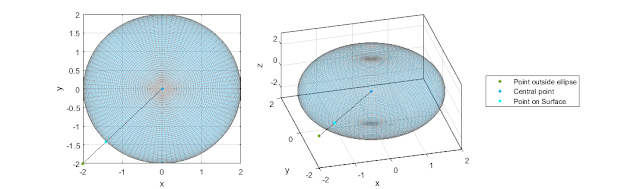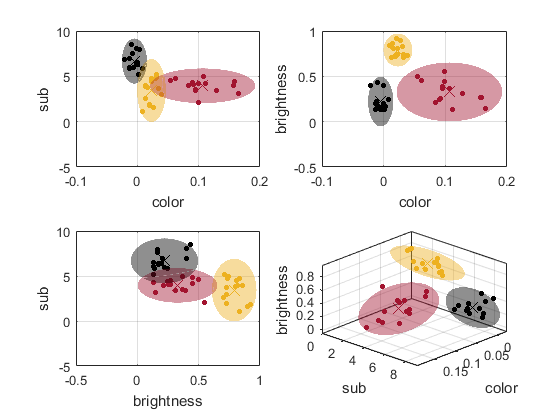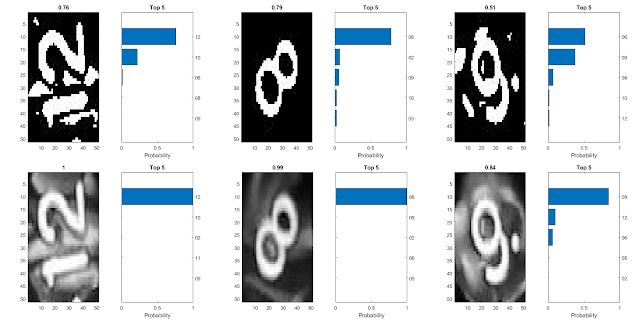## Posts

Es werden Posts vom 2018 angezeigt.

### Matlab: Punkt auf ellipsoidalen Oberflächen(German) This post is focussing on the solution of calculating a point of an ellipsoidal surface in dependency of to angles like in spherical coordinates.
Mode of operation: This function calculates the closest point on an ellipsoidal surface from an point outside or inside the body in respect to its center. It needs the mesh data of the ellipsoidal body X, Y, Z and the point, where the point with direct connection to the center should be found on the surface. The output parameters are [point, phi, the] = PointOnSurfaceAnalytic(X, Y, Z, x, y, z); the surface point and the angles phi and theta of the ellipsoidal equations. It can be downloaded here.

xa+yb+zc2=1
If we consider 𝝋 to be between the x and y axis and
𝝑 be between the y and z axis it leads to:
y=x tan 𝝋
z=y tan 𝝑
Substitute this in the ellipsoidal equation one gets: ya tan 𝝋+yb+y tan 𝝑c2=1
solve this for y gives:
y=11a tan 𝝋+1b+tan 𝝑c2
so one can calculate x, …

### Matlab: Klassifizierung(German) This post describes a Matlab function which classifies images dependent on shape color and so on to differentiate between images like bananas and apples.
Mode of operation: In this function different attributes of images are calculated in values to classify images and seperate them in 2D or 3D. Classification methodes are 'color', 'color2' and 'colorhex' for different color classifications and 'brightness' for the brightness value 'shape', 'std', 'mean', 'cov' and 'sub' are different shape conditions. This function can be downloaded on MathWorks. Following figure shows the classification of apples, bananas and black berries.

It is seen in three dimensions it is clearly classified. Most of the mathematical algortihm are based on magnitude gradient maps to whether calculate shape or substructures. Following figure shows the different input images and their gradient map. In source code give be…

### Matlab: Convolutional Netzwerk für Würfel Nummer Erkennung(English) This post describes a Matlab function which uses a 7 layer convolutional network for dice number identification (numbers 1-12). It calls for the earlier proposed image processing methode shown in this post.
Mode of operation: Two methodes were tested. The dice as shown in previous post is just a tiny part of the whole image. With image processing, the dice itself is excluded and saved in a new folder sorted by number. They are loaded in "digitData". The it is splitted in trainigs set (4/5) and test set (1/5 of alle images). All in all I have 996 images and I tested the not processed images and the binare tresholded images, which suprisingly showed a higher accuracy for the non processed images. An all in all accuracy of 99.6% in the training set and 99.2% in the test set was achieved around 5% higher than for the binare images. In this image the value of accuracy is seen what the convolutional net predicts. A good improvement is seen in non processed …